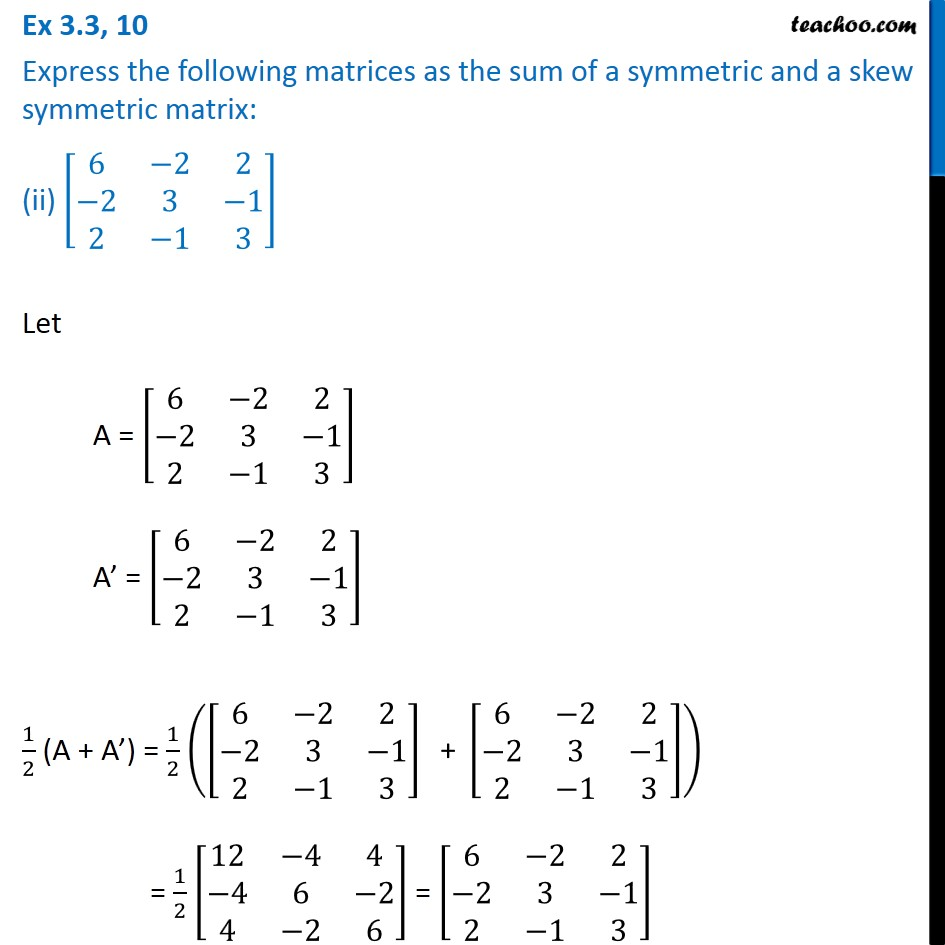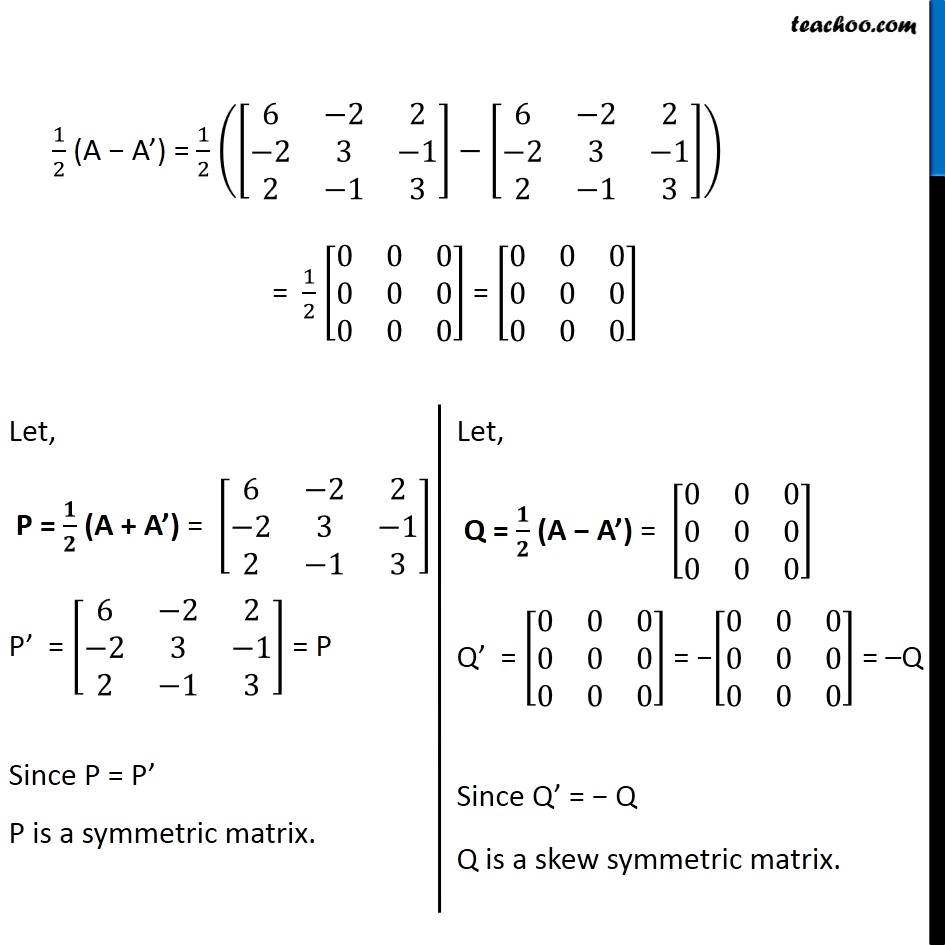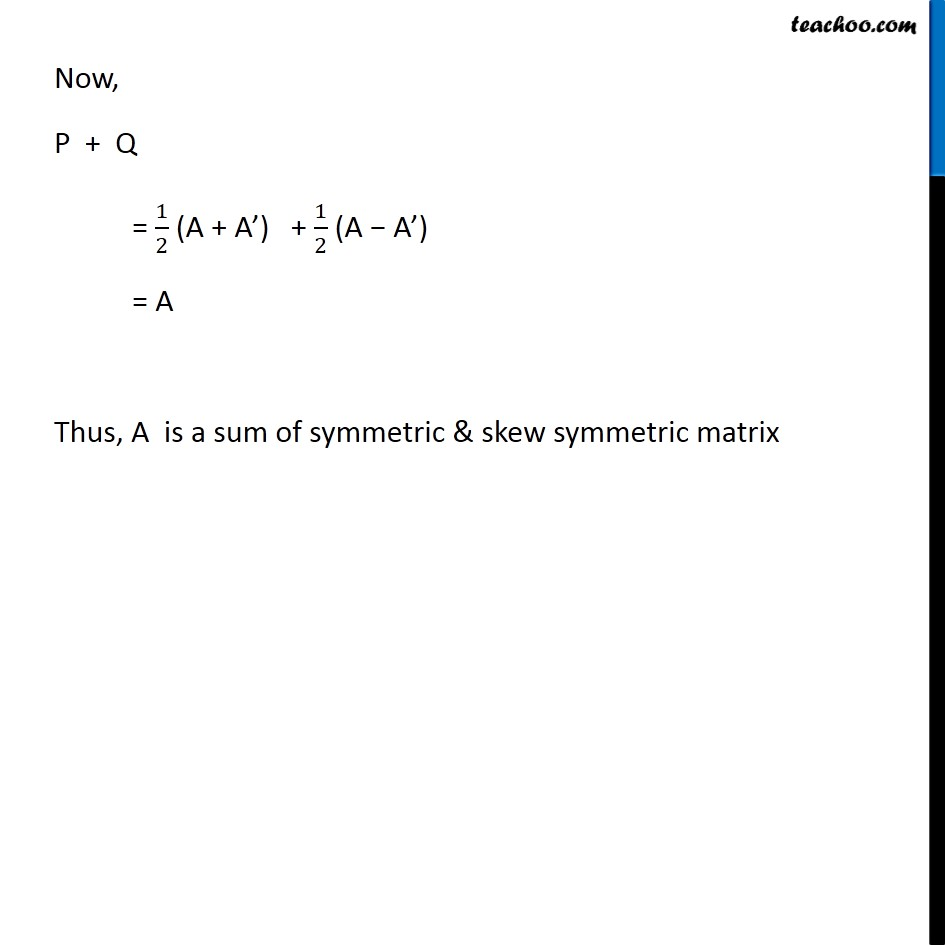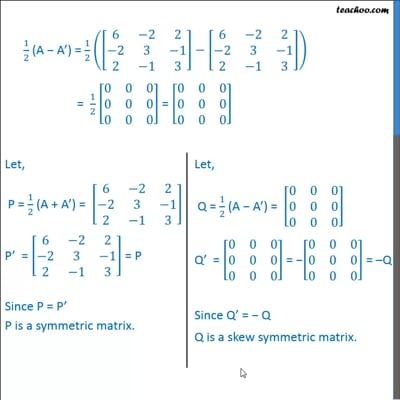Ex 3.3

Chapter 3 Class 12 Matrices
Serial order wiseThis video is only available for Teachoo black users

Introducing your new favourite teacher - Teachoo Black, at only ₹83 per month

### Transcript

Ex 3.3, 10 Express the following matrices as the sum of a symmetric and a skew symmetric matrix: (ii) [■8(6&−2&2@−2&3&−1@2&−1&3)] Let A = [■8(6&−2&2@−2&3&−1@2&−1&3)] A’ = [■8(6&−2&2@−2&3&−1@2&−1&3)] 1/2 (A + A’) = 1/2 ([■8(6&−2&2@−2&3&−1@2&−1&3)]" + " [■8(6&−2&2@−2&3&−1@2&−1&3)]) = 1/2 [■8(12&−4&4@−4&6&−2@4&−2&6)] = [■8(6&−2&2@−2&3&−1@2&−1&3)] 1/2 (A − A’) = 1/2 ([■8(6&−2&2@−2&3&−1@2&−1&3)]−[■8(6&−2&2@−2&3&−1@2&−1&3)]) = 1/2 [■8(0&0&0@0&0&0@0&0&0)] = [■8(0&0&0@0&0&0@0&0&0)] Let, P = 𝟏/𝟐 (A + A’) = [■8(6&−2&2@−2&3&−1@2&−1&3)] P’ = [■8(6&−2&2@−2&3&−1@2&−1&3)] = P Since P = P’ P is a symmetric matrix. Let, Q = 𝟏/𝟐 (A − A’) = [■8(0&0&0@0&0&0@0&0&0)] Q’ = [■8(0&0&0@0&0&0@0&0&0)] = −[■8(0&0&0@0&0&0@0&0&0)] = –Q Since Q’ = − Q Q is a skew symmetric matrix. Now, P + Q = 1/2 (A + A’) + 1/2 (A − A’) = A Thus, A is a sum of symmetric & skew symmetric matrix